# Canvas实现直线与圆形的物理运动效果

## 二、几种简单的直线运动

### 匀速直线运动

HTML代码

```<!DOCTYPE html>
<html lang="en">
<meta charset="UTF-8">
<title>canvas匀速直线运动</title>
<body>
<canvas id="canvas">你的浏览器不支持canvas，请跟换其他浏览器试一试</canvas>
<script type="text/javascript" src="script.js"></script>
</body>
</html>```

JS代码

```window.onload=function(){
var canvas=document.getElementById('canvas');
canvas.height=728;
canvas.width=1024;
var context=canvas.getContext('2d');
context.fillStyle='red';
context.beginPath();
context.arc(800,300,30,0,2*Math.PI,true);
context.closePath();
context.fill();
setInterval(function(){
run(context);
}, 50);
};
var speed=0;
var startPoint=800;
function run(cxt){
speed=-7;
cxt.clearRect(0,0,1024,728);
//cxt.top+=speed;
startPoint+=speed;
cxt.beginPath();
cxt.arc(startPoint,300,30,0,2*Math.PI,true);
cxt.closePath();
cxt.fill();
}```PS：这里面画面有点卡顿，是录制的时候软件的因素造成的，直接运行上诉代码是可以看到正常运行的效果

```var speed=0;
var startPoint=800;
function run(cxt){
speed=-7;
cxt.clearRect(0,0,1024,728);
//cxt.top+=speed;
startPoint+=speed;
cxt.beginPath();
cxt.arc(startPoint,300,30,0,2*Math.PI,true);
cxt.closePath();
cxt.fill();
}```

### 匀变速直线运动

HTML代码：

```<!DOCTYPE html>
<html lang="en">
<meta charset="UTF-8">
<title>canvas匀加速直线运动</title>
<body>
<canvas id="canvas">你的浏览器不支持canvas，请跟换其他浏览器试一试</canvas>
<script type="text/javascript" src="script.js"></script>
</body>
</html>```

JavaScript代码：

```window.onload=function(){
var canvas=document.getElementById('canvas');
canvas.height=728;
canvas.width=1024;
var context=canvas.getContext('2d');
context.fillStyle='red';
context.beginPath();
context.arc(800,300,30,0,2*Math.PI,true);
context.closePath();
context.fill();
setInterval(function(){
run(context);
}, 50);
};
var v0=0;//初始速度
var a=0;//加速度
var v=0;//变化的速度
var time=0;//时间
var x=0;//位移量
var startPoint=800;//起始点
// V=V0+at
// x=v0t+1/2at^2
// v^2-V^2=2ax
function run(cxt){
time+=0.05;
a=10;
x=-(0.5*a*(time*time));//位移公式代入
startPoint+=x;
cxt.clearRect(0,0,1024,728);
cxt.beginPath();
cxt.arc(startPoint,300,30,0,2*Math.PI,true);
cxt.closePath();
cxt.fill();
}```## 三、简单的曲线运动的实现

### 1、匀速圆周运动```<!DOCTYPE html>
<html lang="en">
<meta charset="UTF-8">
<title>canvas实现圆周运动</title>
<body>
<canvas id="canvas">你的浏览器不支持canvas，请跟换其他浏览器试一试</canvas>
<script type="text/javascript" src="script.js"></script>
</body>
</html>```

```window.onload=function(){
var canvas=document.getElementById('canvas');
canvas.height=728;
canvas.width=1024;
var context=canvas.getContext('2d');
drawNotChange(context);
context.fillStyle='blue';
context.beginPath();
context.arc(500,550,30,0,2*Math.PI,true);
context.closePath();
context.fill();
setInterval(function(){
run(context);
}, 50);
};
var time=0;//定义运动的执行次数
function run(cxt){

cxt.clearRect(0,0,1024,728);
drawNotChange(cxt);
cxt.save();//将当前以左上角坐标为(0,0)的上下文环境进行保存，这样是为了在接下来中要进行画布偏移后，可以进行还原当前的环境
cxt.translate(500,400);
cxt.rotate(time*8*Math.PI/180);//设定每次旋转的度数
cxt.fillStyle='blue';
cxt.beginPath();
cxt.arc(0,150,30,0,2*Math.PI,false);
cxt.closePath();
cxt.fill();
cxt.restore();//将当前为(500,400)的点还原为（0,0）,其实在save中就是将上下文环境保存到栈中，在restore下面对其进行还原
time+=1;
}

//绘制不变因素
function drawNotChange(context){
context.fillStyle='red';
context.beginPath();
context.arc(500,400,30,0,2*Math.PI,true);
context.closePath();
context.fill();
context.beginPath();
context.arc(500,400,150,0,2*Math.PI,true);
context.closePath();
context.stroke();
}```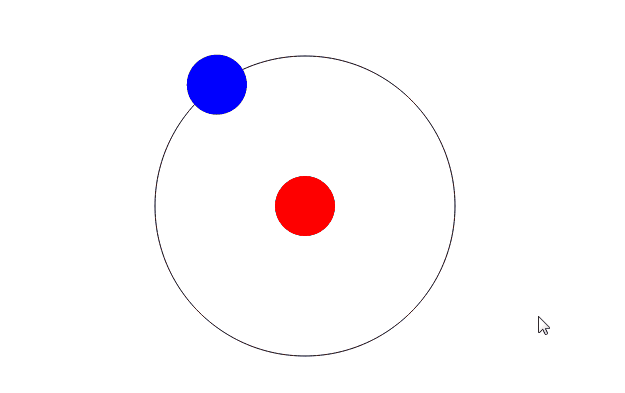### 2、椭圆运动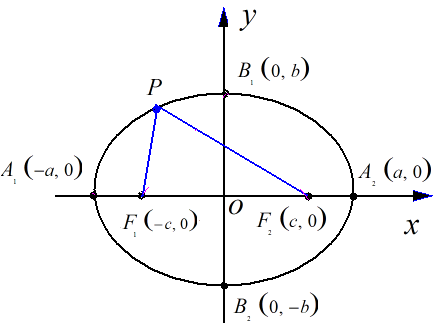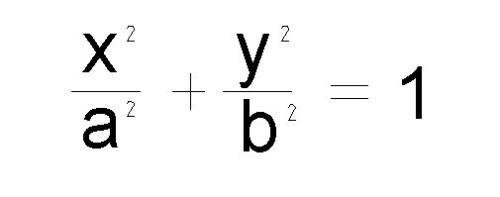x=a+cos(t)

y=b+sin(t)

HTML代码：

```<!DOCTYPE html>
<html lang="en">
<meta charset="UTF-8">
<title>canvas实现椭圆运动</title>
<body>
<canvas id="canvas">你的浏览器不支持canvas，请跟换其他浏览器试一试</canvas>
<script type="text/javascript" src="script.js"></script>
</body>
</html>```

JavaScript代码：

```var a=200,
b=100,
var canvas=document.getElementById('canvas');
canvas.height=668;
canvas.width=1024;
var cxt=canvas.getContext('2d');
cxt.beginPath();
cxt.arc(300,300,10,0,2*Math.PI,true)
cxt.closePath();
cxt.fill();
route(cxt,300,300,200,100);
};

//椭圆路线绘制
function route(context,x,y,a,b){
//max是等于1除以长轴值a和b中的较大者
//i每次循环增加1/max，表示度数的增加
//这样可以使得每次循环所绘制的路径（弧线）接近1像素
var step = (a > b) ? 1 / a : 1 / b;
context.beginPath();
context.moveTo(x + a, y); //从椭圆的左端点开始绘制
for (var i = 0; i < 2 * Math.PI; i += step)
{
//参数方程为x = a * cos(i), y = b * sin(i)，
//参数为i，表示度数（弧度）
context.lineTo(x + a * Math.cos(i), y + b * Math.sin(i));
}
context.closePath();
context.stroke();
}```

椭圆的轨迹的思路是：通过循环，将极小的线段首尾相连，绘制了一个类似于椭圆的一个图像，但是由于线段太过细小导致了我们肉眼看上去就成了一个椭圆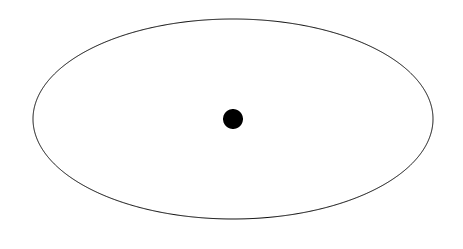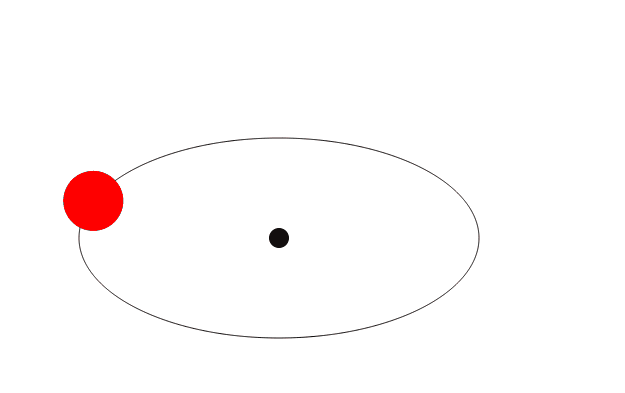HTML代码和上面的例子相同

JavaScript代码如下：

```var a=200,
b=100,
time=0;//循环的次数
var canvas=document.getElementById('canvas');
canvas.height=768;
canvas.width=1024;
var cxt=canvas.getContext('2d');
centerPoint(cxt);
setInterval(function(){
}, 70);
};

//绘制原点
function centerPoint(cxt){
cxt.fillStyle="black";
cxt.beginPath();
cxt.arc(300,300,10,0,2*Math.PI,true)
cxt.closePath();
cxt.fill();
}
//椭圆路线绘制
function route(context,x,y,a,b){
var step = (a > b) ? 1 / a : 1 / b;
context.beginPath();
context.moveTo(x + a, y); //从椭圆的左端点开始绘制
for (var i = 0; i < 2 * Math.PI; i += step)
{
context.lineTo(x + a * Math.cos(i), y + b * Math.sin(i));
}
context.closePath();
context.stroke();
}

//椭圆上小球运动的实现
function arcRoute(context,x,y,a,b,r){
context.clearRect(0,0,1024,768);
route(context,x,x,a,b);
centerPoint(context);
var step = (a > b) ? 1 / a : 1 / b;
context.fillStyle="red";
if(time==0){
context.beginPath();
context.arc(x,y,r,0,2*Math.PI,true);
context.closePath();
context.fill();
}else{
context.beginPath();
context.arc(x+a*Math.cos(time),y+b*Math.sin(time),r,0,2*Math.PI,true);
context.closePath();
context.fill();
}
time+=1;
}```

CSS3制作圆环进度动画效果